The LOGISTIC Procedure

Conditional Logistic Regression

Subsections:

The method of maximum likelihood described in the preceding sections relies on large-sample asymptotic normality for the validity of estimates and especially of their standard errors. When you do not have a large sample size compared to the number of parameters, this approach might be inappropriate and might result in biased inferences. This situation typically arises when your data are stratified and you fit intercepts to each stratum so that the number of parameters is of the same order as the sample size. For example, in a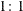matched pairs study with n pairs and p covariates, you would estimate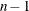intercept parameters and p slope parameters. Taking the stratification into account by "conditioning out" (and not estimating) the stratum-specific intercepts gives consistent and asymptotically normal MLEs for the slope coefficients. See Breslow and Day (1980) and Stokes, Davis, and Koch (2012) for more information. If your nuisance parameters are not just stratum-specific intercepts, you can perform an exact conditional logistic regression .

Computational Details

For each stratum h,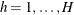, number the observations as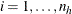so that hi indexes the ith observation in stratum h. Denote the p covariates for the hith observation as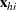and its binary response as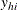, and let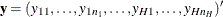,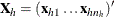, and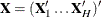. Let the dummy variables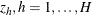, be indicator functions for the strata (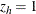if the observation is in stratum h), and denote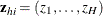for the hith observation,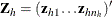, and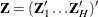. Denote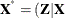) and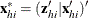. Arrange the observations in each stratum h so that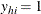for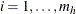, and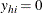for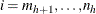. Suppose all observations have unit frequency.

Consider the binary logistic regression model written as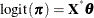where the parameter vector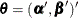consists of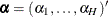,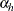is the intercept for stratum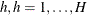, andis the parameter vector for the p covariates.

From the section Determining Observations for Likelihood Contributions, you can write the likelihood contribution of observation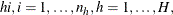as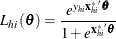wherewhen the response takes Ordered Value 1, andotherwise.

The full likelihood is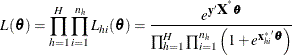Unconditional likelihood inference is based on maximizing this likelihood function.

When your nuisance parameters are the stratum-specific intercepts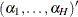, and the slopesare your parameters of interest, "conditioning out" the nuisance parameters produces the conditional likelihood (Lachin, 2000)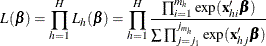where the summation is over all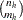subsets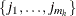of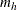observations chosen from the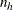observations in stratum h. Note that the nuisance parameters have been factored out of this equation.

For conditional asymptotic inference, maximum likelihood estimates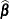of the regression parameters are obtained by maximizing the conditional likelihood, and asymptotic results are applied to the conditional likelihood function and the maximum likelihood estimators. A relatively fast method of computing this conditional likelihood and its derivatives is given by Gail, Lubin, and Rubinstein (1981) and Howard (1972). The optimization techniques can be controlled by specifying the NLOPTIONS statement.

Sometimes the log likelihood converges but the estimates diverge. This condition is flagged by having inordinately large standard errors for some of your parameter estimates, and can be monitored by specifying the ITPRINT option. Unfortunately, broad existence criteria such as those discussed in the section Existence of Maximum Likelihood Estimates do not exist for this model. It might be possible to circumvent such a problem by standardizing your independent variables before fitting the model.

Regression Diagnostic Details

Diagnostics are used to indicate observations that might have undue influence on the model fit or that might be outliers. Further investigation should be performed before removing such an observation from the data set.

The derivations in this section use an augmentation method described by Storer and Crowley (1985), which provides an estimate of the "one-step" DFBETAS estimates advocated by Pregibon (1984). The method also provides estimates of conditional stratum-specific predicted values, residuals, and leverage for each observation. The augmentation method can take a lot of time and memory.

Following Storer and Crowley (1985), the log-likelihood contribution can be written as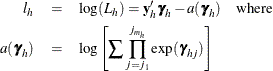and the h subscript on matrices indicates the submatrix for the stratum,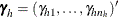, and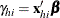. Then the gradient and information matrix are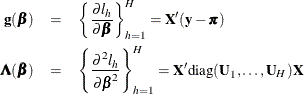where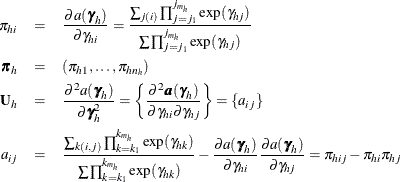and where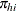is the conditional stratum-specific probability that subject i in stratum h is a case, the summation on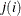is over all subsets from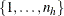of sizethat contain the index i, and the summation on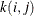is over all subsets fromof sizethat contain the indices i and j.

To produce the true one-step estimate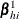, start at the MLE, delete the hith observation, and use this reduced data set to compute the next Newton-Raphson step. Note that if there is only one event or one nonevent in a stratum, deletion of that single observation is equivalent to deletion of the entire stratum. The augmentation method does not take this into account.

The augmented model is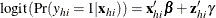where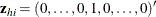has a 1 in the hith coordinate, and use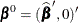as the initial estimate for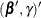. The gradient and information matrix before the step are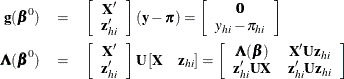Inserting the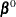and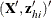into the Gail, Lubin, and Rubinstein (1981) algorithm provides the appropriate estimates of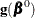and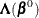. Indicate these estimates with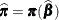,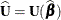,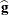, and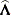.

DFBETA is computed from the information matrix as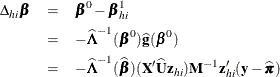where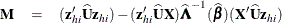For each observation in the data set, a DFBETA statistic is computed for each parameter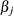,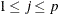, and standardized by the standard error offrom the full data set to produce the estimate of DFBETAS.

The estimated leverage is defined as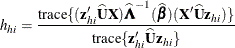This definition of leverage produces different values from those defined by Pregibon (1984); Moolgavkar, Lustbader, and Venzon (1985); Hosmer and Lemeshow (2000); however, it has the advantage that no extra computations beyond those for the DFBETAS are required.

The estimated residuals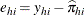are obtained from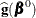, and the weights, or predicted probabilities, are then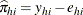. The residuals are standardized and reported as (estimated) Pearson residuals: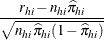where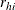is the number of events in the observation and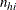is the number of trials.

The STDRES option in the INFLUENCE option computes the standardized Pearson residual: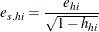For events/trials MODEL statement syntax, treat each observation as two observations (the first for the nonevents and the second for the events) with frequencies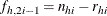and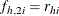, and augment the model with a matrix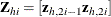instead of a single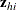vector. Writing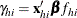in the preceding section results in the following gradient and information matrix: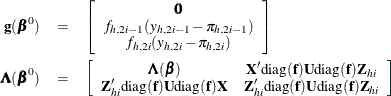The predicted probabilities are then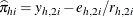, while the leverage and the DFBETAS are produced fromin a fashion similar to that for the preceding single-trial equations.